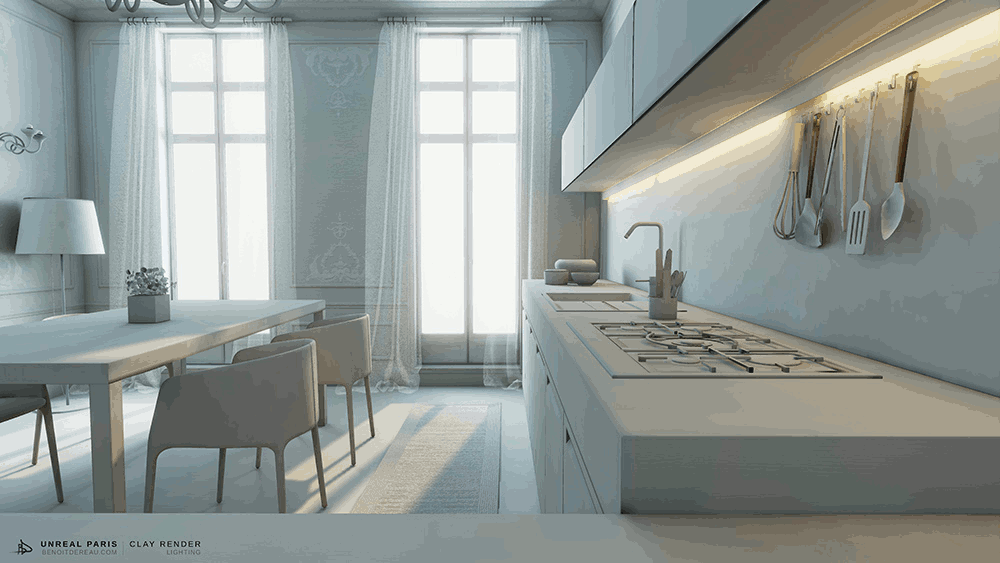# Chapter （1）

，已经被证明不存在初等原函数，这样，就无法用Newton-Leibniz公式，只能另想办法。# 光### 什么是光栅化？什么是光栅？### 2.Geometry几何阶段### 3.Rasterizer光栅器### 但是

#### 不得不承认# Chapter（2）

1979年，TurnerWhitted在光线投射的基础上，加入光与物体表面的交互，是光线在物体表面沿着反射，折射以及散射方式上继续传播，直到与光源相交这一方法后来也被称为经典光线跟踪方法、递归式光线追踪（Recursive Ray Tracing）方法，或 Whitted-style 光线跟踪方法。

by浅墨

### 原理：

#### 我们如果能看到一个物体的某个点 这个点必然反射/折射了光线### Sphere / Ray intersection (给出光线和球的表达式 求相交)

#### Intersection

(1)

or### Illumination Equation(光照方程)

$I{a}K{a}$为递归元素

#### 1. （环境光）$I{amb}=k{a}I_{a}$

$I{a}$是环境光的强度
$k {a}$代表表面环境光反射率在0-1之间

#### 2. （漫反射）$I{diff}=K{d}I{p}cos\left (\theta \right )=K{d}I{p}\left (\vec{N}\cdot \vec{L} \right )+k{a}I_{a}$

$K_{d}$为表面漫反射率

$I_{p}$为点光源强度

$\vec{N}$为表面法向量

$\vec{L}$为入射光方向#### 3. （高光Phong Model）$I{spec}=K{s}I{p}\cos^{n}\left( \phi \right)=K{s}I_{p}\left( \vec{R}\cdot\vec{V} \right)^{n}$$K_{s}$代表表面高光反射率

$I_{p}$代表之前的点光源强度

n代表高光反射参数 设绝对高光镜面（即反射所有光线）为无穷### Snell’s Law(折射定律)

#### 有了这段伪代码我们算是能够在一定程度上了解了光线追踪的过程 但是并不直观 也并不易懂 光线追踪的内核• 对复杂几何网格的追踪，
• 对含有不同材质，纹理的追踪，
• BRDF双向反射分布函数
• 焦散